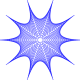# Function Composition

Here’s a paraphrase of a project I’ve assigned to algebra and precalculus classes over the years.  I initially found it years ago in Student Research Projects in Calculus by Marcus Cohen, et al.

Given$f(x)=\frac{1}{x}$ and$g(x)=1-x$.  Functions f and g can be composed with themselves and each other in many ways, but only a finite number of unique functions will result.

• How many functions can result from these compositions?
• Give an algebraic form of each unique function and convince me that you know you have found them all.

That’s it.

I typically allow them to work in small groups of 2 or 3 to test and refine their arguments.  Following are three different successful approaches my students have used over the years.  I’d love to hear of any other approaches.

SOLTUION ALERT! — Don’t read any further if you want to solve this problem on your own.

METHOD 1:  Composition Chains

Ignoring subtle domain differences, most discover$f(f(x))=g(g(x))=x$.  Some then realize that in any random composition sequence of any number of fs and gs, whenever two of the same function are side-by-side in the composition, those letters “cancel out.”  They conclude from this that any new functions after f, g, and$f(f(x))=g(g(x))=x$ must result from alternating fs and gs.  Generating the function list from there gives:

1.$\displaystyle f(x)=\frac{1}{x}$
2.$\displaystyle g(f(x))=1-\frac{1}{x}=\frac{x-1}{x}$
3.$\displaystyle f(g(f(x)))=\frac{x}{x-1}$
4.$\displaystyle g(f(g(f(x))))=1-\frac{x}{x-1}=\frac{-1}{x-1}$
5.$\displaystyle f(g(f(g(f(x)))))=1-x=g(x)$
6.$\displaystyle g(f(g(f(g(f(x))))))=x$
7.$\displaystyle f(g(f(g(f(g(f(x)))))))=\frac{1}{x}=f(x)$

But the last line repeats the first, so there are only 6 functions possible.  Starting with g produces the same chain in a different order.

METHOD 2:  Charts

The next most commonly submitted approach begins with a chart with f and g on the first row and compositions of each in successive rows.  When a previously identified function is encountered, that branch of the chart can be considered terminated as the compositions of that function have already been charted.  As every new function is composed in every way possible, when no new functions appear, you know you have found them all.

As with METHOD 1, compositions in this chart are computed by applying f or g to the existing functions, and not the other way around.  Only 6 unique functions are found in the chart.METHOD 3:  Circles

A few years ago, one group produced the following argument.  It is equivalent to the chart approach in METHOD 2 with the$f(f(x))=g(g(x))=x$ recognition of METHOD 1.  This approach more elegantly captures the cycling nature of the functions.  Because the circle is closed, the only functions are the six shown.

The function listed between each arrow pairing is the function applied to the existing expression.Technology, Algebra, and other Implications

A colleague at my school, LG, once said there are only two parts to every math problem:  1) Show that your answer is correct, and 2) Show that no other answers are correct.  Historically, my students have been 100% convinced that there are only six functions long before they think of a way to prove there are only six.   This project requires students to use some level of creativity to establish the second point–an organizational skill I fear we don’t target often enough in pre-collegiate mathematics.

Once the basics of function composition are understood, this project grants a tremendous amount of initial success to students.  Their errors tend to be overstatements of the number of unique functions (most typically 7 or 8), and almost always because they fail to identify different forms of functions h, k, and sometimes j from METHODS 2 and 3.  Sometimes I have permitted CAS for the project, but even there, students are required to recognize equivalent forms.  As noted below, a CAS can give different algebraic forms of an expression, depending on how it is derived.  Precious few understand their CAS functionality well enough to attempt the Boolean comparison I used in the last step to obtain$k(x)$.So, permitting technology on this project typically has eased student concerns, but it still doesn’t do all of the work for them.

As an extension, I’ve sometimes asked students to state the domains of the functions they derive.  This is a bit of a thorny question as the domains of each function depend on the order of the compositions used to derive them, and as METHOD 3 suggests, there are an infinite number of ways to arrive at each of the six functions.  Most students end up stating the domains of the functions according to the composition order they used to find each function.  I keep waiting for a group to see and describe the composition order dependency, but that hasn’t happened yet.  Even so, I’ve found this to be a great project to inspire mathematical reasoning.  I hope some of you find something in it for your classes.

### 2 responses to “Function Composition”

1.danpearcymaths.wordpress.com

Great Investigation! I thoroughly agree on your approach to get students to convince themselves of this. Will definately use this at some point in teaching – Thanks

2.Dave Radcliffe (@daveinstpaul)

Very nice. The circle diagram is called a Cayley graph, and it is a very important tool in geometric group theory.